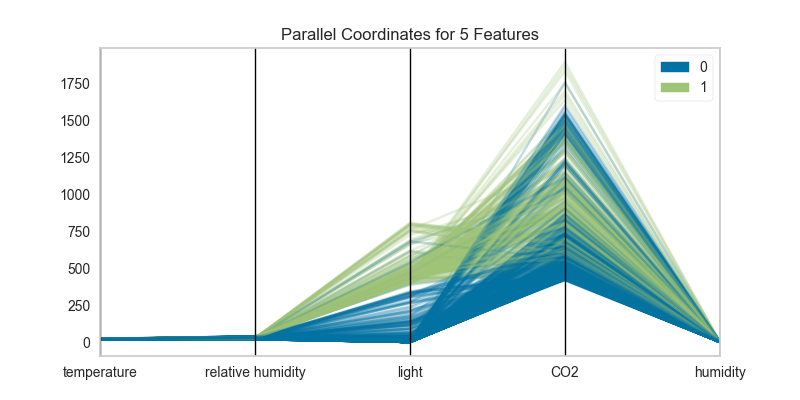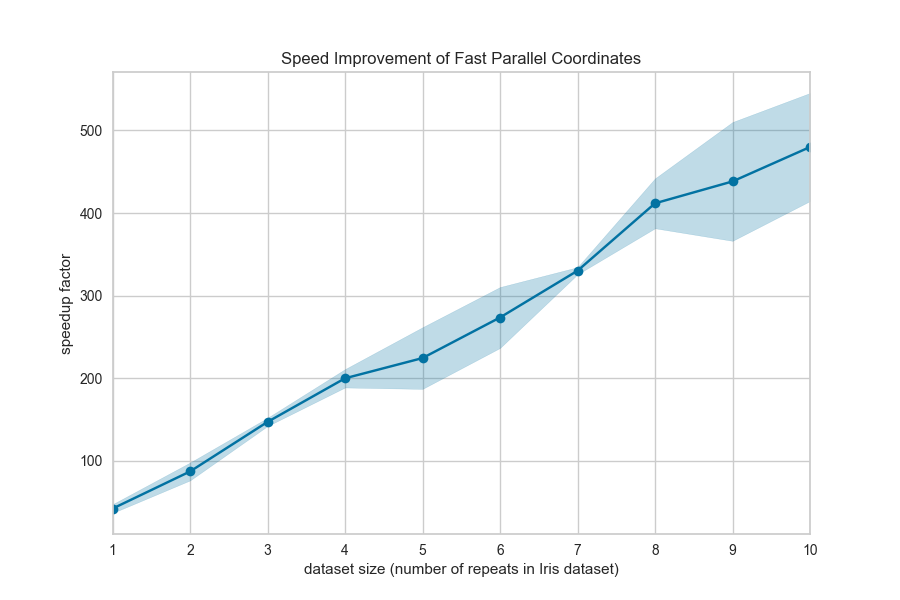# Parallel Coordinates¶

Parallel coordinates is multi-dimensional feature visualization technique where the vertical axis is duplicated horizontally for each feature. Instances are displayed as a single line segment drawn from each vertical axes to the location representing their value for that feature. This allows many dimensions to be visualized at once; in fact given infinite horizontal space (e.g. a scrolling window), technically an infinite number of dimensions can be displayed!

Data scientists use this method to detect clusters of instances that have similar classes, and to note features that have high variance or different distributions. We can see this in action after first loading our occupancy classification dataset:

# Load the classification data set

# Specify the features of interest and the classes of the target
features = [
"temperature", "relative humidity", "light", "C02", "humidity"
]
classes = ["unoccupied", "occupied"]

# Extract the instances and target
X = data[features]
y = data.occupancy


The visualization can be drawn with either the ParallelCoordinates visualizer or using the parallel_coordinates quick method:

from yellowbrick.features import ParallelCoordinates

# Instantiate the visualizer
visualizer = ParallelCoordinates(
classes=classes, features=features, sample=0.5, shuffle=True
)

# Fit and transform the data to the visualizer
visualizer.fit_transform(X, y)

# Finalize the title and axes then display the visualization
visualizer.poof()By inspecting the visualization closely, we can see that the combination of transparency and overlap gives us the sense of groups of similar instances, sometimes referred to as “braids”. If there are distinct braids of different classes, it suggests that there is enough separability that a classification algorithm might be able to discern between each class.

Unfortunately, as we inspect this class, we can see that the domain of each feature may make the visualization hard to interpret. In the above visualization, the domain of the light feature is from in [0, 1600], far larger than the range of temperature in [50, 96]. To solve this problem, each feature should be scaled or normalized so they are approximately in the same domain.

Normalization techniques can be directly applied to the visualizer without pre-transforming the data (though you could also do this) by using the normalize parameter. Several transformers are available; try using minmax, minabs, standard, l1, or l2 normalization to change perspectives in the parallel coordinates as follows:

from yellowbrick.features import ParallelCoordinates

# Instantiate the visualizer
visualizer = ParallelCoordinates(
classes=classes, features=features,
normalize='standard', sample=0.05, shuffle=True,
)

# Fit the visualizer and display it
visualizer.fit_transform(X, y)
visualizer.poof()Now we can see that each feature is in the range [-3, 3] where the mean of the feature is set to zero and each feature has a unit variance applied between [-1, 1] (because we’re using the StandardScaler via the standard normalize parameter). This version of parallel coordinates gives us a much better sense of the distribution of the features and if any features are highly variable with respect to any one class.

## Faster Parallel Coordinates¶

Parallel coordinates can take a long time to draw since each instance is represented by a line for each feature. Worse, this time is not well spent since a lot of overlap in the visualization makes the parallel coordinates less understandable. We propose two solutions to this:

1. Use sample=0.2 and shuffle=True parameters to shuffle and sample the dataset being drawn on the figure. The sample parameter will perform a uniform random sample of the data, selecting the percent specified.
2. Use the fast=True parameter to enable “fast drawing mode”.

The “fast” drawing mode vastly improves the performance of the parallel coordinates drawing algorithm by drawing each line segment by class rather than each instance individually. However, this improved performance comes at a cost, as the visualization produced is subtly different; compare the visualizations in fast and standard drawing modes below:As you can see the “fast” drawing algorithm does not have the same build up of color density where instances of the same class intersect. Because there is only one line per class, there is only a darkening effect between classes. This can lead to a different interpretation of the plot, though it still may be effective for analytical purposes, particularly when you’re plotting a lot of data. Needless to say, the performance benefits are dramatic:## API Reference¶

Implementation of parallel coordinates for multi-dimensional feature analysis.

class yellowbrick.features.pcoords.ParallelCoordinates(ax=None, features=None, classes=None, normalize=None, sample=1.0, random_state=None, shuffle=False, color=None, colormap=None, alpha=None, fast=False, vlines=True, vlines_kwds=None, **kwargs)[source]

Bases: yellowbrick.features.base.DataVisualizer

Parallel coordinates displays each feature as a vertical axis spaced evenly along the horizontal, and each instance as a line drawn between each individual axis. This allows you to detect braids of similar instances and separability that suggests a good classification problem.

Parameters: ax : matplotlib Axes, default: None The axis to plot the figure on. If None is passed in the current axes will be used (or generated if required). features : list, default: None a list of feature names to use If a DataFrame is passed to fit and features is None, feature names are selected as the columns of the DataFrame. classes : list, default: None a list of class names for the legend If classes is None and a y value is passed to fit then the classes are selected from the target vector. normalize : string or None, default: None specifies which normalization method to use, if any Current supported options are ‘minmax’, ‘maxabs’, ‘standard’, ‘l1’, and ‘l2’. sample : float or int, default: 1.0 specifies how many examples to display from the data If int, specifies the maximum number of samples to display. If float, specifies a fraction between 0 and 1 to display. random_state : int, RandomState instance or None If int, random_state is the seed used by the random number generator; If RandomState instance, random_state is the random number generator; If None, the random number generator is the RandomState instance used by np.random; only used if shuffle is True and sample < 1.0 shuffle : boolean, default: True specifies whether sample is drawn randomly color : list or tuple, default: None optional list or tuple of colors to colorize lines Use either color to colorize the lines on a per class basis or colormap to color them on a continuous scale. colormap : string or cmap, default: None optional string or matplotlib cmap to colorize lines Use either color to colorize the lines on a per class basis or colormap to color them on a continuous scale. alpha : float, default: None Specify a transparency where 1 is completely opaque and 0 is completely transparent. This property makes densely clustered lines more visible. If None, the alpha is set to 0.5 in “fast” mode and 0.25 otherwise. fast : bool, default: False Fast mode improves the performance of the drawing time of parallel coordinates but produces an image that does not show the overlap of instances in the same class. Fast mode should be used when drawing all instances is too burdensome and sampling is not an option. vlines : boolean, default: True flag to determine vertical line display vlines_kwds : dict, default: None options to style or display the vertical lines, default: None kwargs : dict Keyword arguments that are passed to the base class and may influence the visualization as defined in other Visualizers. Attributes ——– n_samples_ : int number of samples included in the visualization object

Notes

These parameters can be influenced later on in the visualization process, but can and should be set as early as possible.

Examples

>>> visualizer = ParallelCoordinates()
>>> visualizer.fit(X, y)
>>> visualizer.transform(X)
>>> visualizer.poof()

NORMALIZERS = {'l1': Normalizer(copy=True, norm='l1'), 'l2': Normalizer(copy=True, norm='l2'), 'maxabs': MaxAbsScaler(copy=True), 'minmax': MinMaxScaler(copy=True, feature_range=(0, 1)), 'standard': StandardScaler(copy=True, with_mean=True, with_std=True)}
draw(X, y, **kwargs)[source]

Called from the fit method, this method creates the parallel coordinates canvas and draws each instance and vertical lines on it.

Parameters: X : ndarray of shape n x m A matrix of n instances with m features y : ndarray of length n An array or series of target or class values kwargs : dict Pass generic arguments to the drawing method
draw_classes(X, y, **kwargs)[source]

Draw the instances colored by the target y such that each line is a single class. This is the “fast” mode of drawing, since the number of lines drawn equals the number of classes, rather than the number of instances. However, this drawing method sacrifices inter-class density of points using the alpha parameter.

Parameters: X : ndarray of shape n x m A matrix of n instances with m features y : ndarray of length n An array or series of target or class values
draw_instances(X, y, **kwargs)[source]

Draw the instances colored by the target y such that each line is a single instance. This is the “slow” mode of drawing, since each instance has to be drawn individually. However, in so doing, the density of instances in braids is more apparent since lines have an independent alpha that is compounded in the figure.

This is the default method of drawing.

Parameters: X : ndarray of shape n x m A matrix of n instances with m features y : ndarray of length n An array or series of target or class values

Notes

This method can be used to draw additional instances onto the parallel coordinates before the figure is finalized.

finalize(**kwargs)[source]

Finalize executes any subclass-specific axes finalization steps. The user calls poof and poof calls finalize.

Parameters: kwargs: generic keyword arguments.
fit(X, y=None, **kwargs)[source]

The fit method is the primary drawing input for the visualization since it has both the X and y data required for the viz and the transform method does not.

Parameters: X : ndarray or DataFrame of shape n x m A matrix of n instances with m features y : ndarray or Series of length n An array or series of target or class values kwargs : dict Pass generic arguments to the drawing method self : instance Returns the instance of the transformer/visualizer